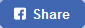Percentage Calculator & Formula - How to Calculate Increase/Decrease - Success Stories Magazine
Press CTRL + D to bookmark this page# Percentage Calculator & Formula

What is % of ?

## How to calculate the percentage of a whole

is what percent of ?
%

## How to calculate percent of increase/decrease between two numbers

What is the percent of increase/decrease
from to ?
%

## What is ___% of ___?

What is 10% of 25?
First convert percent to a decimal 10%/100 = 0.1
Y = 10% × 25 = 0.1 × 25 = 2.5
Therefore, 2.5 is 10% of 25.

## ___ is what percent of ___?

12 is what percent of 40?
Rewrite as 12 is P% of 40
Solve for P%: P% = Y ÷ X
Y ÷ X = 12 ÷ 40 = 0.3
Convert the decimal to percent
P% = 0.3 × 100 = 30%
Therefore, 12 is 30% of 40.

## ___ is ___% of what?

9 is 60% of what?
Rewrite as 9 is 60% of X
Solve for X: X = Y ÷ P%
X = 9 ÷ 60%
Convert percent to decimal
60% ÷ 100 = 0.6
X = 9 ÷ 0.6
X = 15
Therefore, 9 is 60% of 15

## What percent of ___ is ___?

What percent of 27 is 6?
Rewrite as P% of 27 is 6
Solve for P%: P% = Y ÷ X
6 ÷ 27 = 0.2222
Convert decimal to percent
P% = 0.2222 × 100
P% = 22.22%
Therefore, 22.22% of 27 is 6.

## ___% of what is ___?

20% of what is 7?
Rewrite as 20% of X is 7
Solve for X: X = Y ÷ P%
First convert percent to a decimal
20% ÷ 100 = 0.2, then
X = 7 ÷ 20% = 7 ÷ 0.2
X = 35
Therefore, 20% of 35 is 7.

## ___% of ___ is what?

5% of 29 is what?
Rewrite as 5% of 29 is Y?
Solve for Y: Y = P% × X
First convert percent to a decimal
5% ÷ 100 = 0.05
Y = 5% × 29 = 0.05 × 29
Y = 1.45
Therefore, 5% of 29 is 1.45.

## ___ out of what is ___%?

4 out of what is 12%?
Rewrite as 4 out of X is 12%?
Solve for X: X = Y ÷ P%
First convert percent to a decimal
12% ÷ 100 = 0.12, then
X = 4 ÷ 12% = 4 ÷ 0.12
X = 33.3333
Therefore, 4 out of 33.3333 is 12%.

## What out of ___ is ___%?

What out of 25 is 11%?
Rewrite as Y out of 25 is 11%?
Solve for Y: Y = P% × X
First convert percent to a decimal
11% ÷ 100 = 0.11, then
Y = 11% × 25 = 0.11 × 25
Y = 2.75
Therefore, 2.75 out of 25 is 11%.

## ___ out of ___ is what percent?

9 out of 13 is what percent?
Rewrite as 9 out of 13 is P%?
Solve for P%: P% = Y ÷ X
9 ÷ 13 = 0.6923
Convert decimal to percent
0.6923 × 100 = 69.23%
P% = 69.23%
Therefore, 9 out of 13 is 69.23%.

Do not worry about your difficulties in Mathematics. I can assure you mine are still greater.

Albert Einstein

[gtranslate]